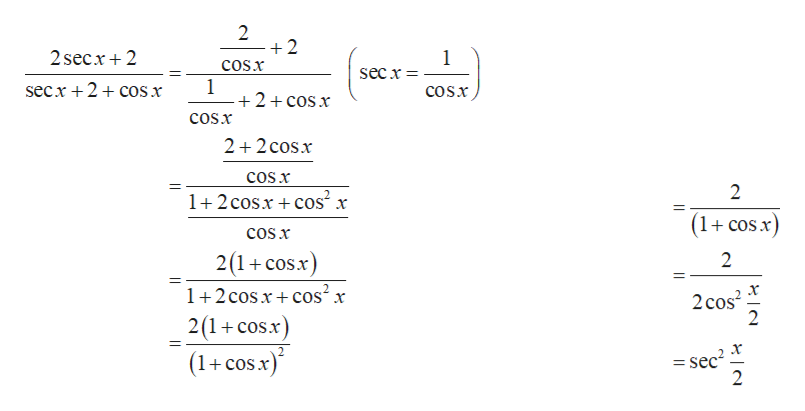# Prove the identity.sec2 (x/2)= 2 sec x + 2/sec x + 2 + cos x

Question
14 views

Prove the identity.

sec(x/2)= 2 sec x + 2/sec x + 2 + cos x

check_circle

Step 1

The given equation is,

Step 2

Consider right hand side of the equation:help_outlineImage Transcriptionclose2 2 2secx2 1 COSX secx 1 2 cos x secx + 2 + сos x cosx COsx 2 2 cosx COS x 2 1+2 cosxcos2 r (1+cos x) coSx 2(1+cosx) 2 1+2 cosrcos2 x 2cos2 2 2(1 cosx 11 (1+cos.x = sec 2 fullscreen
Step 3

Consider left hand side ...

### Want to see the full answer?

See Solution

#### Want to see this answer and more?

Solutions are written by subject experts who are available 24/7. Questions are typically answered within 1 hour.*

See Solution
*Response times may vary by subject and question.
Tagged in

### Trigonometric Ratios